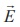Home | | Physics 12th Std | Equi-potential Surface

# Equi-potential Surface

Physics : Electrostatics: Electrostatic Potential and Potential Energy : Equi-potential Surface

Equi-potential Surface

Consider a point charge q located at some point in space and an imaginary sphere of radius r is chosen by keeping the charge q at its center (Figure 1.28(a)). The electric potential at all points on the surface of the given sphere is the same. Such a surface is called an equipotential surface.

An equipotential surface is a surface on which all the points are at the same potential. For a point charge the equipotential surfaces are concentric spherical surfaces as shown in Figure 1.28(b). Each spherical surface is an equipotential surface but the value of the potential is different for different spherical surfaces.For a uniform electric field, the equipotential surfaces form a set of planes normal to the electric field. This is shown in the Figure 1.29.

Properties of equipotential surfaces

(i) The work done to move a charge q between any two points A and B, W = q (VB – VA). If the points A and B lie on the same equipotential surface, work done is zero because VA = VB.

(ii) The electric field is normal to an equipotential surface. If it is not normal, then there is a component of the field parallel to the surface. Then work must be done to move a charge between two points on the same surface. This is a contradiction. Therefore the electric field must always be normal to equipotential surface.Tags : Electrostatics , 12th Physics : Electrostatics
Study Material, Lecturing Notes, Assignment, Reference, Wiki description explanation, brief detail
12th Physics : Electrostatics : Equi-potential Surface | Electrostatics